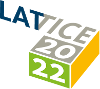#The 39th International Symposium on Lattice Field Theory (Lattice 2022)

Aug 8 – 13, 2022
Hörsaalzentrum Poppelsdorf
Europe/Berlin timezone

## Fourier acceleration in strongly-interacting linear sigma models

Aug 9, 2022, 8:00 PM
1h
Poster Presentation Algorithms (including Machine Learning, Quantum Computing, Tensor Networks)

### Speaker

Joshua Swaim (University of Connecticut)

### Description

Fourier acceleration is a technique used in Hybrid Monte Carlo (HMC) simulations to decrease the autocorrelation length. In the weak interaction limit, Fourier acceleration eliminates the problem of critical slowing down. In this work, we show that by properly tuning the kinetic term in HMC simulations, Fourier acceleration can be applied effectively to a strongly interacting $\phi^4$ theory. We use this algorithm to study the linear sigma model with a large coupling constant and in the spontaneous symmetry breaking phase. We find that Fourier acceleration leads to a reduced autocorrelation length and faster thermalization. In addition, we find a method to make use of the tuned kinetic term in the Fourier-accelerated HMC to further reduce the statistical error of many observables. There are several on-going efforts that try to apply Fourier acceleration to non-Abelian gauge theories like QCD. We think some techniques developed in this work can help the application of Fourier acceleration to QCD.

### Primary authors

Joshua Swaim (University of Connecticut) Luchang Jin (University of Connecticut)